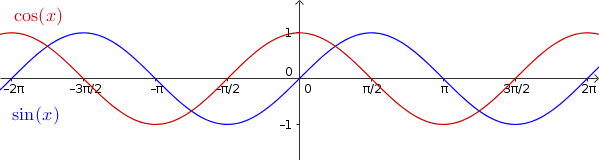Math Sine and cosine function Sine and cosine wave

# Sine and cosine wave

The simplest sine function has the function equation $y=\sin(x)$. The graph is called the sine wave.

The simplest cosine function has the function equation $y=\cos(x)$. The graph is called the cosine wave.

!

### Remember

To specify the angle $\alpha$ (see unit circle) not in degrees on the coordinate system, it is often converted to radian.

$180^\circ\mathrel{\hat=}\pi$

The concrete formula for converting is:

$x=\alpha\cdot\frac{\pi}{180^\circ}$i

### Properties

• The range $W=[-1,1]$ and the domain $D=\mathbb{R}$ (real numbers)
• The amplitude (maximum deflection) is 1
• The periode (repetition) is $2\pi$